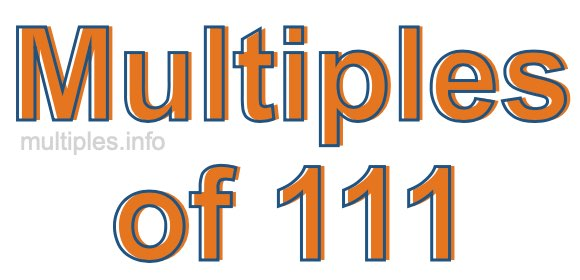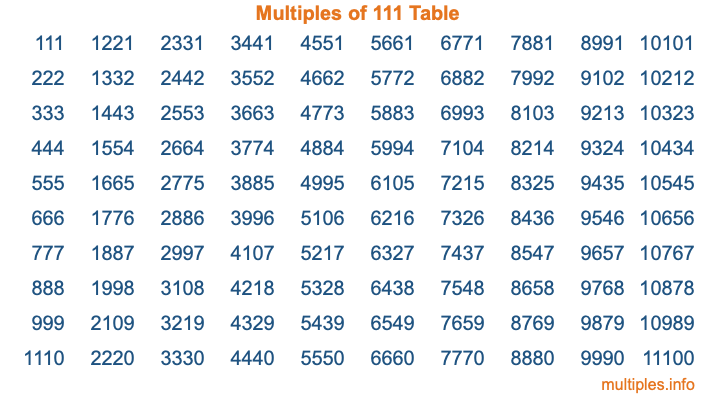Multiples of 111Welcome to the Multiples of 111 page. Here we will first teach you everything you will ever need to know about the multiples of 111, and then give you a study guide summary of everything we taught you to make sure you remember it all. Use this page to look up facts and learn information about the multiples of 111. This page will make you a multiples of one hundred eleven expert!

Definition of Multiples of 111
Multiples of 111 are all the numbers that when divided by 111 equal an integer. Each of the multiples of 111 are called a multiple. A multiple of 111 is created by multiplying 111 by an integer.

Therefore, to create a list of multiples of 111, you start with 1 multiplied by 111, then 2 multiplied by 111, then 3 multiplied by 111, and so on for as long as you want. Thus, the list of the first five multiples of 111 is 111, 222, 333, 444, and 555. To see a larger list of multiples of 111, see the printable image of Multiples of 111 further down on this page. We also have a category where you can choose any nth multiple of 111.

Multiples of 111 Checker
The Multiples of 111 Checker below checks to see if any number of your choice is a multiple of 111. In other words, it checks to see if there is any number (integer) that when multiplied by 111 will equal your number. To do that, we divide your number by 111. If the the quotient is an integer, then your number is a multiple of 111.

Is  a multiple of 111?

Least Common Multiple of 111 and ...
A Least Common Multiple (LCM) is the lowest multiple that two or more numbers have in common. This is also called the smallest common multiple or lowest common multiple and is useful to know when you are adding our subtracting fractions. Enter one or more numbers below (111 is already entered) to find the LCM.

Check out our LCM Calculator if you need more details about the Least Common Multiple or if you need the LCM for different numbers for adding and subtraction fractions.

nth Multiple of 111
As we stated above, 111 is the first multiple of 111, 222 is the second multiple of 111, 333 is the third multiple of 111, and so on. Enter a number below to find the nth multiple of 111.

th multiple of 111

Multiples of 111 vs Factors of 111
111 is a multiple of 111 and a factor of 111, but that is where the similarities end. All postive multiples of 111 are 111 or greater than 111. All positive factors of 111 are 111 or less than 111.

Below is the beginning list of multiples of 111 and the factors of 111 so you can compare:

Multiples of 111: 111, 222, 333, 444, 555, etc.

Factors of 111: 1, 3, 37, 111

As you can see, the multiples of 111 are all the numbers that you can divide by 111 to get a whole number. The factors of 111, on the other hand, are all the whole numbers that you can multiply by another whole number to get 111.

It's also interesting to note that if a number (x) is a factor of 111, then 111 will also be a multiple of that number (x).

Multiples of 111 vs Divisors of 111
The divisors of 111 are all the integers that 111 can be divided by evenly. Below is a list of the divisors of 111.

Divisors of 111: 1, 3, 37, 111

The interesting thing to note here is that if you take any multiple of 111 and divide it by a divisor of 111, you will see that the quotient is an integer.

Multiples of 111 Table
Below is an image of the first 100 multiples of 111 in a table. The table is in chronological order, column by column. The first column has the first ten multiples of 111, the second column has the next ten multiples of 111, and so on.The Multiples of 111 Table is also referred to as the 111 Times Table or Times Table of 111. You are welcome to print out our table for your studies.

Negative Multiples of 111
Although not often discussed or needed in math, it is worth mentioning that you can make a list of negative multiples of 111 by multiplying 111 by -1, then by -2, then by -3, and so on, to get the following list of negative multiples of 111:

-111, -222, -333, -444, -555, etc.

Multiples of 111 Summary
Below is a summary of important Multiples of 111 facts that we have discussed on this page. To retain the knowledge on this page, we recommend that you read through the summary and explain to yourself or a study partner why they hold true.

There are an infinite number of multiples of 111.

A multiple of 111 divided by 111 will equal a whole number.

111 divided by a factor of 111 equals a divisor of 111.

The nth multiple of 111 is n times 111.

The largest factor of 111 is equal to the first positive multiple of 111.

111 is a multiple of every factor of 111.

111 is a multiple of 111.

A multiple of 111 divided by a divisor of 111 equals an integer.

111 divided by a divisor of 111 equals a factor of 111.

Any integer times 111 will equal a multiple of 111.

Multiples of a Number
Here you can get the multiples of another number, all with the same attention to detail as we did for multiples of 111 on this page.

Multiples of
Multiples of 112
Did you find our page about multiples of one hundred eleven educational? Do you want more knowledge? Check out the multiples of the next number on our list!